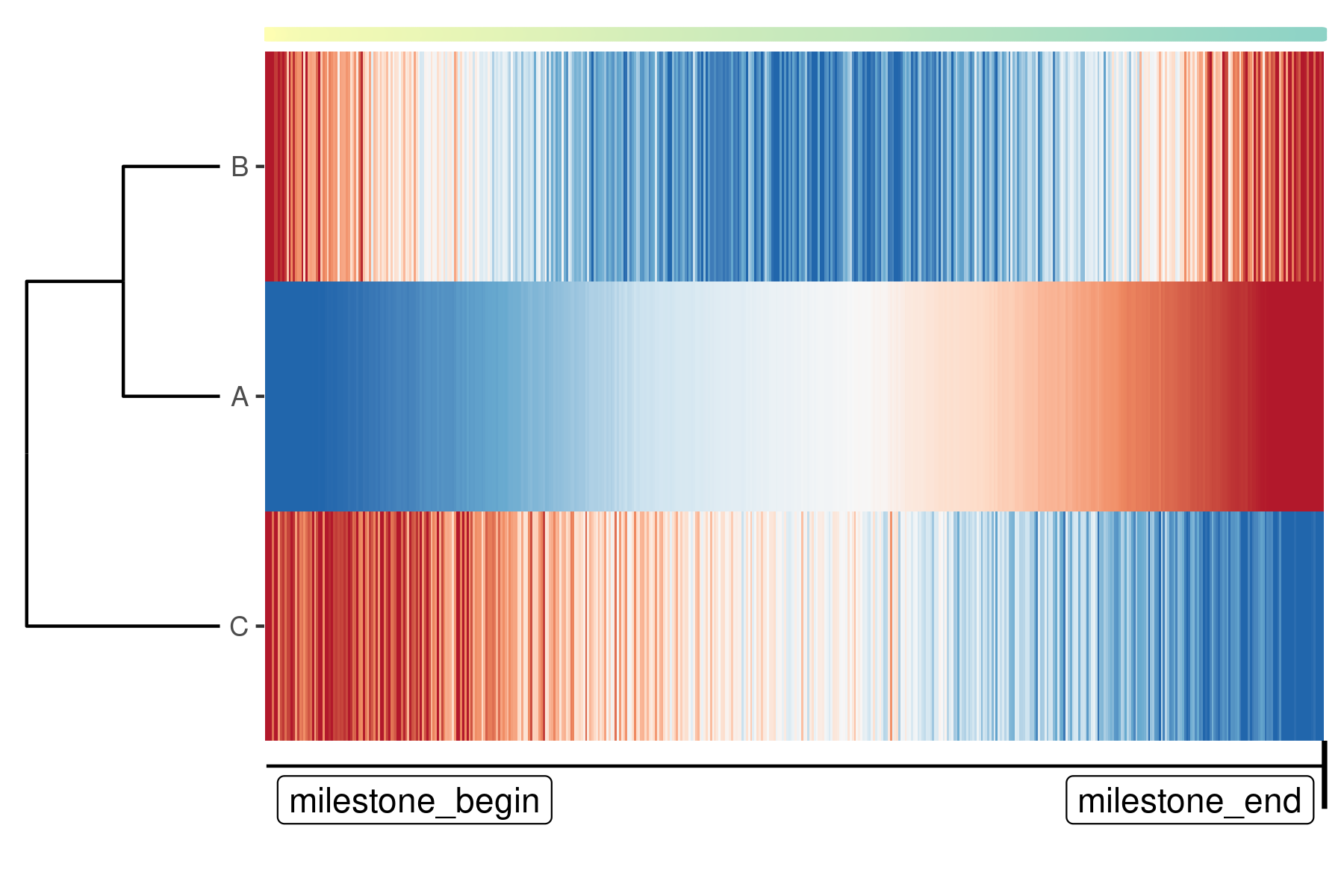Creating a TI method: Script

2021-03-23

library(dynwrap)

An alternative to wrapping a script inside R, is to wrap it using an external script. Because this does not provide any dependency management, this is not really useful for method end-users, but rather as a way to easily develop a TI method and to ultimately step up towards containerised wrapping.

Similarly as a wrapper written in R, you’ll need to provide both a definition (= a definition.yml) and a way to run the methods (= a script).

definition.yml

The definition has the same hierarchical structure as used by the definition() function. A minimal example

definition.yml
method:
id: comp_1

parameters:
- id: component
default: 1
type: integer
distribution:
type: uniform
lower: 1
upper: 10
description: The nth component to use

wrapper:
input_required: expression
input_optional: start_id

You can use our template definition.yml to see all the different possibilities of what can be included in the definition: https://github.com/dynverse/dynmethods/tree/master/template_container . It may also be useful to check out some of the wrappers in dynmethods, e.g.: https://github.com/dynverse/ti_paga/blob/master/definition.yml

Running script

A running script reads in the data, creates a trajectory, and again writes the trajectory to a file. To do the loading and writing tasks, we provide helper libraries for R and python: dyncli and dynclipy.

The wrapper script will typically have the following structure:

• A shebang, such as #!/usr/bin/env Rscript or #!/usr/bin/env python
• A call to dyncli(py) to load in the data, such as dataset <- dyncli::main() or dataset = dynclipy.main()
• All you need to infer the trajectory
• Constructing the trajectory model using any of the wrapping methods
• A call to dyncli(py) to write the data, such as dyncli::write_output(trajectory) or trajectory.write_output()

A minimal example script for R:

run.R
#!/usr/bin/env Rscript

dataset <- dyncli::main()

library(dynwrap)
library(dplyr)
library(stats)
library(dyncli)

# infer trajectory
pca <- prcomp(dataset$expression) pseudotime <- pca$x[, dataset$parameters$component]

# flip pseudotimes using start_id
if (!is.null(dataset$priors$start_id)) {
if (mean(pseudotime[start_id]) > 0.5) {
pseudotime <- 1 - pseudotime
}
}

# build trajectory
trajectory <- wrap_data(cell_ids = rownames(dataset$expression)) %>% add_linear_trajectory(pseudotime = pseudotime) # save output write_output(trajectory, dataset$output)

Make sure this script is executable!

chmod +x run.R

and for Python:

run.py
#!/usr/bin/env python

import dynclipy
dataset = dynclipy.main()

import pandas as pd
import sklearn.decomposition

# infer trajectory
pca = sklearn.decomposition.PCA()
dimred = pca.fit_transform(dataset['expression'])
pseudotime = pd.Series(
dimred[:, dataset['parameters']['component']-1],
index = dataset['expression'].index
)

# build trajectory
trajectory = dynclipy.wrap_data(cell_ids = dataset['expression'].index)

# save output
trajectory.write_output(dataset['output'])

Testing it out

method <- create_ti_method_definition("definition.yml", "run.R")
dataset <- dynwrap::example_dataset
trajectory <- infer_trajectory(dataset, method(), verbose = TRUE)

If you have dynplot installed, you can also plot the trajectory:

library(dynplot)
# for now, install from github using:
# remotes::install_github("dynverse/dynplot")
plot_graph(trajectory)
plot_heatmap(trajectory, expression_source = dataset\$expression)Interactive development

With the debug parameter, it is possible to enter the script interactively. This makes it easier to develop TI method as you can just load in the input data, and code!

trajectory <- infer_trajectory(dataset, method(), debug = TRUE)

Making your method available for other users

Wrapping a method inside a script does not have any dependency management, and is therefore only meant for development purposes. To deploy your method to other users, check out the containerisation tutorial!

Wrapping a method without dyncli(py)

While using dyncli to load and save the data is the most straightforward when using R and python, it’s also possible to skip these packages and do all the work yourself. Briefly you have to:

• Parse the arguments provided by dynwrap yourself, e.g. --dataset input.h5.
• Read in the hdf5 files generated by dynwrap
• Write the output file in the correct hdf5 format